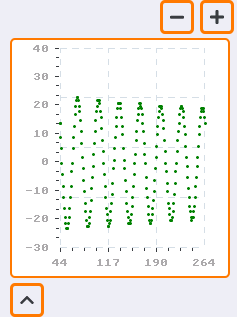# Last tick line on chart isn't drawn

## Description

I have noticed that the last major tickline isn’t drawn on any of the axis’. Is this a bug?

Simulator

### What do you want to achieve?

I would like to have the last tick lines drawn on my axis’

### What have you tried so far?

I have messed a bit around in `lv_chart.c ` but it hasnt changed anything.

## Code to reproduce

I don’t think its a problem with my code but… you never know of course.
I hope I included all the necessary parts. This is quickly put together from the “actual code”

``````/*You code here*/
char yValueList = {""};
char xValueList = {""};
char yValue      = {""};
char xValue      = {""};

lv_chart_ext_t * chart_ext;
lv_chart_axis_cfg_t * x_axis_ext;
lv_chart_axis_cfg_t * y_axis_ext;

static lv_obj_t* chart              = NULL;

void func(void) {
chart = lv_chart_create(lv_scr_act(), NULL);
lv_obj_set_style_local_size         (chart, LV_CHART_PART_SERIES, LV_STATE_DEFAULT, 1);
lv_obj_set_style_local_line_width   (chart, LV_CHART_PART_SERIES, LV_STATE_DEFAULT, 0);
lv_obj_set_size                     (chart, 220, 240);
lv_obj_align                        (chart, Container, LV_ALIGN_CENTER, 0, 0);
lv_chart_set_type                   (chart, LV_CHART_TYPE_LINE);   //Show lines and points too
lv_chart_set_update_mode            (chart, LV_CHART_UPDATE_MODE_SHIFT);
lv_chart_set_point_count            (chart, POINTCOUNT);
lv_chart_set_range                  (chart, -30, 40);
lv_obj_set_event_cb                 (chart, Scrollable_cb);
//lv_chart_cursor_t *c1 = lv_chart_add_cursor(chart, LV_COLOR_RED, LV_CHART_CURSOR_LEFT);
lv_obj_set_style_local_text_font    (chart, LV_OBJ_PART_MAIN, LV_STATE_DEFAULT, &lv_font_unscii_8);

AxisPlotter(chart, XminData /*this is just integers*/, XmaxData /*this is just integers*/![image|237x317], 3, 4, chart_ext->ymax[LV_CHART_AXIS_PRIMARY_Y], chart_ext->ymin[LV_CHART_AXIS_PRIMARY_Y], (int) (chart_ext->ymax[LV_CHART_AXIS_PRIMARY_Y]-chart_ext->ymin[LV_CHART_AXIS_PRIMARY_Y])/10, 3, 3, 2);
}

void AxisPlotter(lv_obj_t * chart, int xMin, int xMax, int xTickLength, int xMinorLine, int yMin, int yMax, int yTickLength, int yMinorLine, int hDiv, int vDiv) {
if (chart == NULL) return;
y_axis_ext = &chart_ext->y_axis;
x_axis_ext = &chart_ext->x_axis;
y_axis_ext->options = LV_CHART_AXIS_DRAW_LAST_TICK;
x_axis_ext->options = LV_CHART_AXIS_INVERSE_LABELS_ORDER;

lv_chart_refresh(chart);

strcpy(yValueList,"");
strcpy(xValueList,"");
strcpy(yValue,"");
strcpy(xValue,"");
for (int y = 0; y <= yTickLength; y++) {
sprintf(yValue, "%i", (int)(((yMax - yMin) * y / yTickLength) + yMin));
strcat(yValueList, yValue);
if (y < yTickLength) {
strcat(yValueList, "\n");
}
}
for (int x = 0; x <= xTickLength; x++) {
sprintf(xValue, "%i", (int)(((xMax - xMin) * x / xTickLength) + xMin));
strcat(xValueList, xValue);
if (x < xTickLength) {
strcat(xValueList, "\n");
}
}

//strcat(xValueList, "0");
//strcat(yValueList, "0");

lv_chart_set_y_tick_length(chart, 4, 3);
lv_chart_set_y_tick_texts(chart, yValueList, yMinorLine, NULL);
lv_chart_set_div_line_count(chart,hDiv,vDiv);
lv_chart_set_x_tick_texts(chart, xValueList, xMinorLine, NULL);
lv_chart_set_x_tick_length(chart, 4, 3);
``````

## Screenshot and/or videoAnd for anyone interested in an answer (since you must have worked this out by now) the last argument in `lv_chart_set_x/y_tick_texts` should be set to `LV_CHART_AXIS_DRAW_LAST_TICK`.